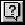# Constructing an Array of Polyline Points

Constructing an Array of Polyline Points

The last issue to deal with is how to transform the individual point variables—p1, p2, p3, and p4—into the format required for the vla-addLightweightpolyline function. First, get some help on the topic.

To obtain information on a function

1. Choose the Help button on the VLISP toolbar.2. Enter vla-addLightweightpolyline in the Enter Item Name dialog box, and choose OK. (The Help system is not case sensitive, so do not worry about how you capitalize the function name.)

Help states that AddLightWeightPolyline requires you to specify the polyline vertices as an array of doubles in the form of a variant. Here is how Help describes this parameter:

`The array of 2D WCS coordinates specifying the vertices of the polyline. At least two points (four elements) are required for constructing a lightweight polyline. The array size must be a multiple of 2.`

A variant is an ActiveX construct that serves as a container for various types of data. Strings, integers, and arrays can all be represented by variants. The variant stores data along with the information identifying the data.

So far, you have four points, each in the format (x, y, z). The challenge is to convert these four points into a list of the following form:

`(x1 y1 x2 y2 x3 y3 x4 y4)`

The append function takes multiple lists and concatenates them. To create a list of the four points in the proper format for the ActiveX function, you can use the following expression:

`(setq polypoints (append (3dPoint->2dPoint p1)`
`                              (3dPoint->2dPoint p2)`
`                              (3dPoint->2dPoint p3)`
`                              (3dPoint->2dPoint p4)))`

Writing the 3dPoint->2dPoint function four times is a bit cumbersome. You can reduce the code further by using the mapcar and apply functions. When selected, mapcar executes a function on individual elements in one or more lists, and apply passes a list of arguments to the specified function. The resulting code looks like the following:

`(setq polypoints (apply 'append (mapcar '3dPoint->2dPoint `
`(list p1 p2 p3 p4))))`

Before the call to mapcar, the list of points is in this form:

`((x1 y1 z1) (x2 y2 z2) (x3 y3 z3) (x4 y4 z4))`

After mapcar you have a list of points in the following form:

`((x1 y1) (x2 y2) (x3 y3) (x4 y4))`

And finally, after applying the append function on the list returned from mapcar, you end up with the following:

`(x1 y1 x2 y2 x3 y3 x4 y4)`